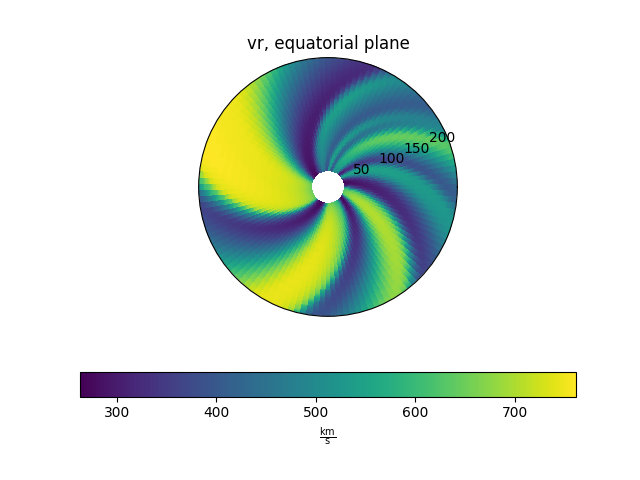# Plotting equatorial slices

This example shows how to plot slices in the equatorial plane from a MAS model output.

```from psipy.model import MASOutput
from psipy.data import sample_data

import matplotlib.pyplot as plt
```

Next, load a set of MAS output files. You will need to change this line to point to a folder with MAS files in them.

```mas_path = sample_data.mas_helio()
model = MASOutput(mas_path)
```

Each MAS model contains a number of variables. The variable names can be accessed using the `.variables` attribute.

```print(model.variables)
```

Out:

```['br', 'rho', 'vr']
```

Set parameters for plotting. This line will give us a horizontal errorbar underneath the plots.

```cbar_kwargs = {'orientation': 'horizontal'}
```

Plot the slices

```ax = plt.subplot(projection='polar')
model['vr'].plot_equatorial_cut(ax=ax, cbar_kwargs=cbar_kwargs)

plt.show()
```Total running time of the script: ( 0 minutes 0.212 seconds)

Gallery generated by Sphinx-Gallery